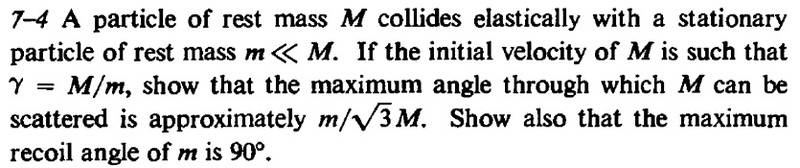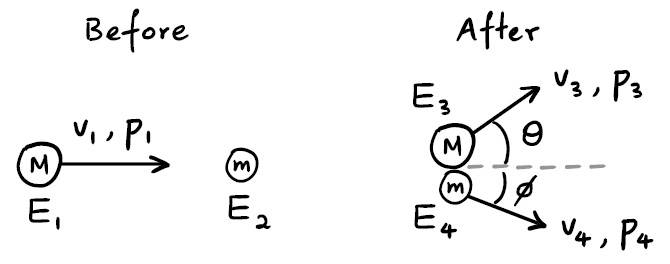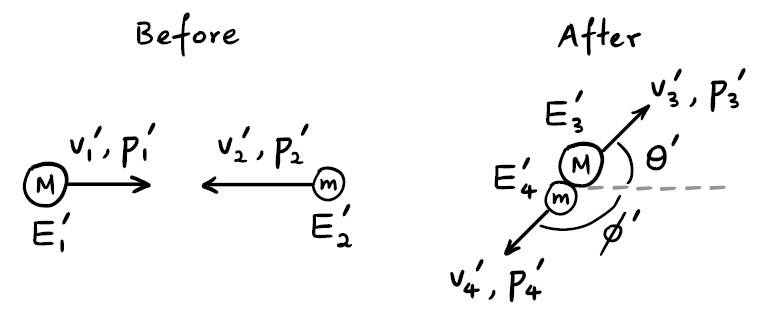# Max angle of deflection in a relativistic elastic collision

• redoopi

## Homework Statement## Homework Equations

Conservation of linear momentum
Conservation of energy
Energy-momentum relationship: ##E^2-(m_0c^2)^2=c^2p^2##
Lorentz transformation of energy: ##p=\gamma(v)(p'+vE'/c^2)##
##\mathbf{p}=\gamma(v)m_0v##, ##E=\gamma(v)m_0c^2##

## The Attempt at a Solution

My attempt to the first question:

In laboratory frame ##S##:In a zero-momentum frame ##S'## moving with velocity ##v## relative to ##S##:Components of ##p_3'## are:
$$p_{3x}'=p_3'\cos\theta' \tag{1}$$
$$p_{3y}'=p_3'\sin\theta' \tag{2}$$
By Lorentz transformations for energy and momentum,
$$p_{3x}=\gamma(v)(p_{3x}'+vE_3'/c^2) \tag{3}$$
$$p_{3y}=p_{3y}' \tag{4}$$
Since
$$E_3'=\gamma(v_3')Mc^2 \tag{5}$$
$$p_3'=\gamma(v_3')Mv_3' \tag{6}$$
Substitute (6) into (5),
$$E_3'=p_3'c^2/v_3' \tag{7}$$
Substitute (1) and (7) into (3),
$$p_{3x}=\gamma(v)p_3'(\cos\theta'+v/v_3') \tag{8}$$
Substitute (2) into (4),
$$p_{3y}=p_3'\sin\theta' \tag{9}$$
So the angle of deflection of mass $M$ is given by
$$\tan\theta=\frac{p_{3y}}{p_{3x}}=\frac{\sin\theta'}{\gamma(v)(\cos\theta'+v/v_3')} \tag{10}$$
If we can resolve ##\gamma(v)## and ##v_3'##, then we can take the derivative of ##\tan\theta## with respect to ##\theta'## and obtain its maximum value.

But I am facing two difficulties:

(1) How to obtain ##\gamma(v)##? My attempt:
$$v_2'=-v \tag{11}$$
$$v_1'=v_1-v \tag{12}$$
$$\gamma(v_1)=M/m \tag{13} \quad \text{(given in the question)}$$
$$\gamma(v_2')=\gamma(-v)=\gamma(v) \tag{14}$$
Since $S'$ is a zero-momentum frame,
$$\gamma(v_1')Mv_1'=\gamma(v_2')mv_2' \tag{15}$$
Substitute (11) - (14) into (15),
$$\gamma(v_1-v)M(v_1-v)=-\gamma(v)mv \tag{16}$$
Now we can solve for ##v## and then calculate ##\gamma(v)##, but the calculation is very complicated.

(2) How to obtain ##v_3'##? Apply energy-momentum relationship on mass ##M## in ##S'## after collision:
$$(E_3')^2-(Mc^2)^2=(cp_3')^2 \tag{17}$$
Now we have equation (7) and (17) for the three variables ##E_3'##, ##p_3'## and ##v_3'##. We need one more relationship to solve for ##v_3'##.

Besides, it will take too much effort to solve the above equations. I guess there should be a simpler approach. Do you have any idea?

Note 1 : The author only briefly mentioned 4-vectors, so we are not supposed to use it to solve this problem.

Note 2: Another approach is as follows:
$$\mathbf{p}_1=\mathbf{p}_3+\mathbf{p}_4 \tag{18}$$
$$E_1+mc^2=E_3+E_4 \tag{19}$$
$$E_1=\gamma(v_1)Mc^2 \tag{20}$$
$$E_1^2-(Mc^2)^2=c^2p_1^2 \tag{21}$$
$$E_3^2-(Mc^2)^2=c^2p_3^2 \tag{22}$$
$$E_4^2-(mc^2)^2=c^2p_4^2 \tag{23}$$
By (18),
$$(\mathbf{p}_1-\mathbf{p}_3)\cdot(\mathbf{p}_1-\mathbf{p}_3)=\mathbf{p}_4\cdot\mathbf{p}_4 \tag{24}$$
$$p_1^2-2p_1p_3\cos\theta+p_3^2=p_4^2 \tag{25}$$
Substitute (13) and (19) - (23) into (25), take derivative of ##\cos\theta## with respect to ##p_3##, set it to zero, then we can find the value of ##p_3## that gives the maximum value of ##\theta##. But the computation is very complicated.

Note 3: A general expression for ##\theta## can be found on p.309 of Goldstein's Classical Mechanics. It seems that the answer in the question is incorrect.

#### Attachments

Equation (17) is wrong. Equation (12) is wrong. I don’t see the point of (7) because
Now we have equation (7) and (17) for the three variables
if you think combining 7 and (the corrected) 17 will lead to anything but a trivial identity... then I disagree.
This is what happens when you work with every variable: you get lost in blind alleys. You only need two of {mass, energy, momentum, speed, gamma-factor} to determine all the others; try not lose sight of the physics amongst the identities.
(1) How to obtain γ(v)?
You defined v to be the velocity of the center of mass. How would you find that classically?

I have a question (for anybody) about this question. “Elastic collision” only implies the sum of the masses is conserved. Is there any reason to assume each mass is individually conserved (other than to make the problem solvable)?

Last edited:
@redoopi I agree that the answer as given in the statement of the problem is incorrect. I believe the answer is ##\theta_{max} = \sin^{-1}\frac{m}{M}## for any initial speed of ##M## (as long as ##M > m##).

So, for ##M \gg m##, ##\theta_{max} \approx \frac{m}{M}##

Your method outlined at the end of your post (Note 2) will work. I don't see a way to avoid the tedious calculation. It took me about 2 pages of algebra. If you want to try working it through, here are some intermediate expressions that I found (with ##c = 1##): $$\cos \theta = \frac{E_3 \left( E_1+m \right) - E_1 m - M^2}{p_1 p_3}$$
##\theta_{max}## occurs when $$E_3 = \frac{M^2(E_1+m)}{M^2 + E_1m}$$ The corresponding value of ##p_3## is
$$p_3 = \frac{p_1 M \sqrt{ M^2-m^2 }}{M^2+E_1m}$$

Is this a problem from a textbook? If so, can you tell us which textbook?

•mccoy1
Equation (17) is wrong. Equation (12) is wrong. I don’t see the point of (7) because
if you think combining 7 and (the corrected) 17 will lead to anything but a trivial identity... then I disagree.
This is what happens when you work with every variable: you get lost in blind alleys. You only need two of {mass, energy, momentum, speed, gamma-factor} to determine all the others; try not lose sight of the physics amongst the identities.
Equation (12) (and hence (16)) is wrong, I should have used relativistic velocity addition (I found it difficult to get out of the habit of using Galilean velocity addition).
But why is equation (17) wrong? The rest energy (##Mc^2##) is an energy-momentum invariant, i.e. ##(E^2)-(cp)^2=(E'^2)-(cp')^2=(Mc^2)^2##.
Equation (7) and (17) contain three variables (##E_3',p_3',v_3'##). So we still need one more equation to solve them.
You defined v to be the velocity of the center of mass. How would you find that classically?
I have a question (for anybody) about this question. “Elastic collision” only implies the sum of the masses is conserved. Is there any reason to assume each mass is individually conserved (other than to make the problem solvable)?
I didn't assume each mass is individually conserved. ##M## and ##m## represent the rest masses. The relativistic masses are ##\gamma M## and ##\gamma m##.

@redoopi I agree that the answer as given in the statement of the problem is incorrect. I believe the answer is ##\theta_{max} = \sin^{-1}\frac{m}{M}## for any initial speed of ##M## (as long as ##M > m##).

So, for ##M \gg m##, ##\theta_{max} \approx \frac{m}{M}##

Your method outlined at the end of your post (Note 2) will work. I don't see a way to avoid the tedious calculation. It took me about 2 pages of algebra. If you want to try working it through, here are some intermediate expressions that I found (with ##c = 1##): $$\cos \theta = \frac{E_3 \left( E_1+m \right) - E_1 m - M^2}{p_1 p_3}$$
##\theta_{max}## occurs when $$E_3 = \frac{M^2(E_1+m)}{M^2 + E_1m}$$ The corresponding value of ##p_3## is
$$p_3 = \frac{p_1 M \sqrt{ M^2-m^2 }}{M^2+E_1m}$$

Is this a problem from a textbook? If so, can you tell us which textbook?

The textbook is "Special Relativity" by A.P. French.

I finally took the time to do the calculations. Surprisingly it is not as tedious as I thought. Here are my calculations:
\begin{align}
p_1^2 &= (\gamma^2-1)M^2c^2 \\
p_1 &= \sqrt{(\gamma^2-1)}Mc \\
E_4=E_1+mc^2-E_3 &= \gamma Mc^2+mc^2-\sqrt{c^2p_3^2+M^2c^4} \\
&=(\gamma M+m)c^2-c\sqrt{p_3^2+M^2c^2} \\
E_4^2 &= (\gamma M+m)^2c^4-2(\gamma M+m)c^3\sqrt{p_3^2+M^2c^2}+p_3^2c^2+M^2c^4 \\
&= (\gamma^2+1)M^2c^4+2\gamma Mmc^4+m^2c^4-2(\gamma M+m)c^3\sqrt{p_3^2+M^2c^2}+p_3^2c^2 \\
p_4^2 &= (\gamma^2+1)M^2c^2+2\gamma Mmc^2-2(\gamma M+m)c\sqrt{p_3^2+M^2c^2}+p_3^2 \\
(\gamma^2-1)M^2c^2-2\sqrt{(\gamma^2-1)}Mcp_3\cos\theta &= (\gamma^2+1)M^2c^2+2\gamma Mmc^2-2(\gamma M+m)c\sqrt{p_3^2+M^2c^2} \\
2\sqrt{(\gamma^2-1)}Mcp_3\cos\theta &= -2M^2c^2-2\gamma Mmc^2+2(\gamma M+m)c\sqrt{p_3^2+M^2c^2} \\
\cos\theta &= \frac{(\gamma M+m)\sqrt{p_3^2+M^2c^2}-M^2c-\gamma Mmc}{\sqrt{(\gamma^2-1)}Mp_3}
\end{align}
Then I use Wolfram Alpha to solve ##d(cos\theta)/dp_3=0## for ##p_3##:
solve d/dp (((g*M+m) sqrt(p^2+M^2*c^2)-M^2*c-g*M*m*c)/(sqrt(g^2-1)*M*p)) = 0 for p
\begin{align}
p_3 &= \frac{Mc\sqrt{\gamma^2-1}\sqrt{M^2-m^2}}{\gamma m+M}
\end{align}
Take ##\gamma=M/m##, we have
\begin{align}
p_3 &= \frac{(M^2-m^2)c}{2m}\approx \frac{M^2c}{2m} \\\\
\cos\theta_{max} &\approx \frac{(M^2+m^2)\sqrt{M^2+4m^2}-4Mm^2}{M^2\sqrt{M^2-m^2}} \\
&\approx 1-2\left(\frac{\sqrt{2}m}{M}\right)^2
\end{align}
Since ##\cos x=1-2\sin^2 (x/2)##, we have
\begin{align}
\sin\frac{\theta_{max}}{2} &\approx \frac{\sqrt{2}m}{M}
\end{align}
Therefore,
\begin{align}
\theta_{max} &\approx \frac{2\sqrt{2}m}{M}
\end{align}

But why is equation (17) wrong? The rest energy (##Mc^2##) is an energy-momentum invariant, i.e. ##(E^2)-(cp)^2=(E'^2)-(cp')^2=(Mc^2)^2##.
Yes, that is correct. In your OP you mixed the mass and momentum terms.

Equation (7) and (17) contain three variables (##E_3',p_3',v_3'##). So we still need one more equation to solve them.
I guess you can say that... In my eyes those 3 variables represent one unknown with zero equations. 7 and 17 are just identities which combine to give more identities.

I didn't assume each mass is individually conserved. ##M## and ##m## represent the rest masses. The relativistic masses are ##\gamma M## and ##\gamma m##.
You did assume that (I believe you have to). When I say mass I mean rest mass. “Elastic collision” means kinetic energy is conserved, which then implies rest energy is conserved, which means that the total (rest) mass is conserved before and after the collision. What I’m wondering is how we known each mass is unchanged. If we had m → m+k and M → M-k it would still be elastic.

The textbook is "Special Relativity" by A.P. French.
That's a well-known, standard text. But I still think that his answer with the ##\sqrt{3}## is wrong.

$$\cos\theta = \frac{(\gamma M+m)\sqrt{p_3^2+M^2c^2}-M^2c-\gamma Mmc}{\sqrt{(\gamma^2-1)}Mp_3}$$

$$p_3 = \frac{Mc\sqrt{\gamma^2-1}\sqrt{M^2-m^2}}{\gamma m+M}$$
OK, these appear to agree with the expressions I listed in my previous post.

Take ##\gamma=M/m##, we have

$$p_3 = \frac{(M^2-m^2)c}{2m}\approx \frac{M^2c}{2m}$$
This is too severe of an approximation if you want your result for ##\cos \theta_{max}## to be accurate to order ##\frac{m^2}{M^2}##. Note that you can write
$$p_3 = \frac{(M^2-m^2)c}{2m} = \frac{M^2c}{2m} \left( 1 - \frac{m^2}{M^2} \right)$$ and you need to keep the factor ##\left( 1 - \frac{m^2}{M^2} \right)##.

That's a well-known, standard text. But I still think that his answer with the ##\sqrt{3}## is wrong.
OK, these appear to agree with the expressions I listed in my previous post.
This is too severe of an approximation if you want your result for ##\cos \theta_{max}## to be accurate to order ##\frac{m^2}{M^2}##. Note that you can write
$$p_3 = \frac{(M^2-m^2)c}{2m} = \frac{M^2c}{2m} \left( 1 - \frac{m^2}{M^2} \right)$$ and you need to keep the factor ##\left( 1 - \frac{m^2}{M^2} \right)##.
By substituting the above expression for ##p_3## and ##\gamma=M/m## into the expression for ##\cos\theta## (equation (10)), we can obtain
$$\cos\theta_{max}=\sqrt{1-\left(\frac{m}{M}\right)^2}$$
and hence ##\sin\theta_{max}=m/M##.

By substituting the above expression for ##p_3## and ##\gamma=M/m## into the expression for ##\cos\theta## (equation (10)), we can obtain
$$\cos\theta_{max}=\sqrt{1-\left(\frac{m}{M}\right)^2}$$
and hence ##\sin\theta_{max}=m/M##.
Looks good. You can show that this result for ##\sin \theta_{max}## does not depend on assuming ##\gamma = \frac{M}{m}##. The only condition is that ##M >m##.

Yes, that is correct. In your OP you mixed the mass and momentum terms.
I don't get it. ##(E_3')^2-(Mc^2)^2=(cp_3')^2## is exactly the form of ##(E'^2)-(cp')^2=(Mc^2)^2##.
You did assume that (I believe you have to). When I say mass I mean rest mass. “Elastic collision” means kinetic energy is conserved, which then implies rest energy is conserved, which means that the total (rest) mass is conserved before and after the collision. What I’m wondering is how we known each mass is unchanged. If we had m → m+k and M → M-k it would still be elastic.
You may read p.169-172 of "Special Relativity" by A.P. French. The idea is like this: In Newtonian mechanics, we know by experiments that a specific type of collisions can be described by the equation ##\alpha_1\mathbf{u}_1+\alpha_2\mathbf{u}_2=\alpha_1\mathbf{v}_1+\alpha_2\mathbf{v}_2##, where the scalars ##\alpha_1## and ##\alpha_2## are intrinsic properties of object 1 and 2. We call that property inertial mass, and we have conservation of linear momentum. We also found that in this case kinetic energy is conserved, and we call this type of collision "perfectly elastic". Now in relativistic mechanics, this simple equation no longer holds. But if we assert conservation of linear momentum, then we must re-define inertial mass (##\alpha_i##) to be ##\gamma## times the inertial mass in Newtonian mechanics (which we now called the rest mass, and is a constant in the equation). So it is experimental facts + definition.
Note: By Keppler 2nd Ed. p.479, the term "mass" refers to relativisitc mass. If you mean "rest mass", you should state it explicitly.

Last edited:
By substituting the above expression for ##p_3## and ##\gamma=M/m## into the expression for ##\cos\theta## (equation (10)), we can obtain
$$\cos\theta_{max}=\sqrt{1-\left(\frac{m}{M}\right)^2}$$
and hence ##\sin\theta_{max}=m/M##.

After all that I'm wondering if this is any different from the result you would obtain in a classical (ie non relativistic) collision for which m << M ?

After all that I'm wondering if this is any different from the result you would obtain in a classical (ie non relativistic) collision for which m << M ?
Good observation. The relativistic result for ##\theta_{max}## does not depend on the initial speed of ##M## when ##m \ll M##. So, the relativistic result for ##\theta_{max}## matches the classical result when ##m \ll M##.

In principle, should it be possible to find an exact expression for maximum angle of deflection for ANY mass M colliding with stationary mass m given just M > m. [so no approximations such as M +- m ≅ M, or sin(m/M) ≅ m/M ] ? For M = m I think the answer is π/2.

In principle, should it be possible to find an exact expression for maximum angle of deflection for ANY mass M colliding with stationary mass m given just M > m. [so no approximations such as M +- m ≅ M, or sin(m/M) ≅ m/M ] ? For M = m I think the answer is π/2.
Yes. For any ##M>m## and for any initial speed of ##M## the result is ##\sin \theta_{max} = m/M##. So, this agrees with the classical result. Also, as you noted, ##\theta_{max} = \pi /2## for ##M = m##.

If ##M \gg m##, then of course you can further simplify to ##\theta_{max} \approx m/M##.

For maximum deflection, the angle between original line of motion (of larger mass) and trajectory of the smaller mass (after collision) given by acos(m/M)/2. Alternatively 180 - (same angle) for angle between original line of motion and line of centres during collision. Does that seem right? Thus far it's an empirical result.